Inflation is the rate of increase in the cost of goods and services over a given period of time.

This example aims to predict inflation from the macroeconomic data of a country.

### Contents

We solve this example with the data science and machine learning platform Neural Designer. To follow this example step by step, you can use the free trial.

## 1. Application type

This is a forecasting project since the variable to predict is the future inflation.

The goal here is to model the inflation rate for the next month from different macroeconomic features of the past three months.

## 2. Data set

The data set contains information to create our model. We need to configure three things:

• Data source.
• Variables.
• Instances.

The data file used for this example is macroeconomics.csv, which contains monthly information about 16 features for 19 years.

The data set includes the following variables:

• date: from January of 2001 to November of 2019.
• reference_rate_NBP: the reference rate of the Central Bank of Poland.
• consumer_price_index: the Consumer Price Index of Poland.
• account_balance: current account balance of Poland in million euros.
• avg_monthly_salary_enterprise: average monthly gross nominal salary in the enterprise sector (growth rate).
• avg_employment_enterprise: average employment in the enterprise sector (growth rate).
• sold_production_industry: total sold production of industry (growth rate).
• price_index_industry: price index of sold production in the industry (growth rate).
• unemployment_rate: registered unemployment rate at the end of the month.
• EURPLN: monthly average of daily closing levels of 1 euro to zloty.
• USDPLN: monthly average of daily closing levels of 1 US dollar to zloty.
• CHFPLN: monthly average of daily closing levels of 1 Swiss franc to zloty.
• WIG20: monthly average of stock market index of the twenty largest companies on the Warsaw Stock Exchange.
• WIG: monthly average of the Warsaw Stock Index.
• WIBOR 3M: monthly average of the Warsaw Interbank Offered Rate.
• core_inflation: inflation excluding food and energy prices.

On the other hand, the instances are divided sequentially into training, selection, and testing subsets, containing 60%, 20%, and 20% of the cases, respectively.

We can calculate the inputs-target correlations. These indicate which macroeconomic factors have the most significant influence on inflation.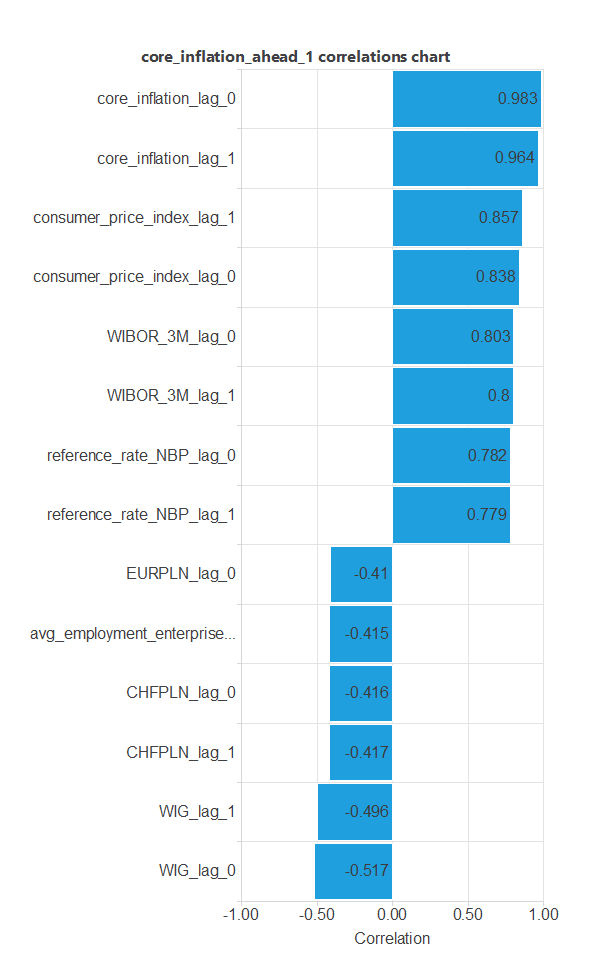In this example, there are a few variables that correlate highly with the target variable. They are WIBOR_3M, consumer_price_index, and reference_rate_NBP.

We can also check the time series charts for these variables.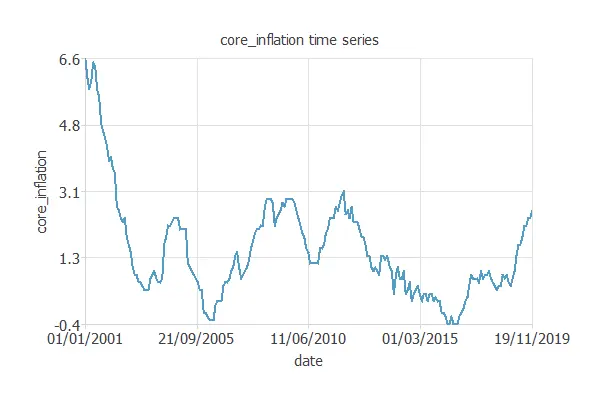Looking at the time series plot for the target variable, we can see that core inflation has been in the same range for the past 15 years.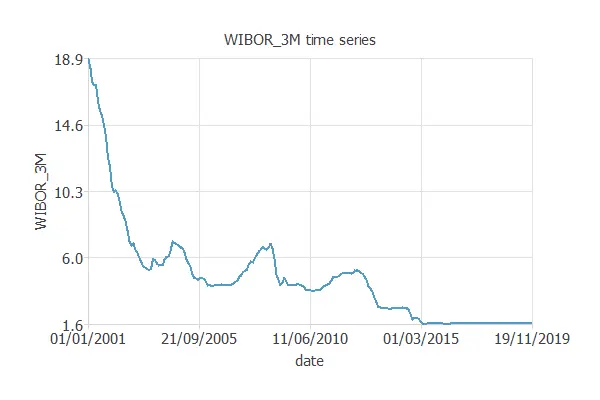We can also look at the WIBOR_3M chart. If we compare the two previous plots, we see the correlation between the two variables.

### 3. Neural network

The next step is to set the neural network parameters. In our case, it is composed of:

• Scaling layer.
• Perceptron layer.
• Probabilistic layer.

We could have also used an LSTM layer.

The mean and standard deviation scaling method has been set for the scaling layer.

Next, we set one perceptron layer with 3 neurons that have the hyperbolic tangent activation function. This layer has 45 inputs, which are the 15 variables of the dataset for three months. The output is one, the core_inflation for the next month.

The neural network for this example can be represented with the following diagram:## 4. Training strategy

The fourth step is to set the training strategy defining what the neural network will learn. A general training strategy for classification is composed of two terms:

• A loss index.
• An optimization algorithm.

The loss index chosen for this problem is the normalized squared error between the outputs from the neural network and the targets in the data set with L1 regularization.

The selected optimization algorithm is the Quasi-Newton method.

The following chart shows how the training error develops with the epochs during the training process.

The final value is training error = 0.00641 NSE and selection error = 0.289 NSE.## 5. Model selection

The objective of model selection is to improve the neural network’s generalization capabilities or, in other words, to reduce the selection error.

First, we perform the neurons selection. We want a model whose complexity is the most appropriate to produce an adequate fit of the data. The optimal value for this example is one neuron.

Next, we will apply an input selection algorithm. This reduces our inputs to six: the consumer_price_index and the core_inflation for the past three months.

The resulting neural network is as follows.With it, the selection error decreases to selection error = 0.0578 NSE. It is a great improvement compared to the previous value.

## 6. Testing analysis

The objective of the testing analysis is to validate the generalization performance of the trained neural network. To validate a forecasting technique, we need to compare the values provided by this technique to the observed values. We can use linear regression analysis as the standard testing method for these projects.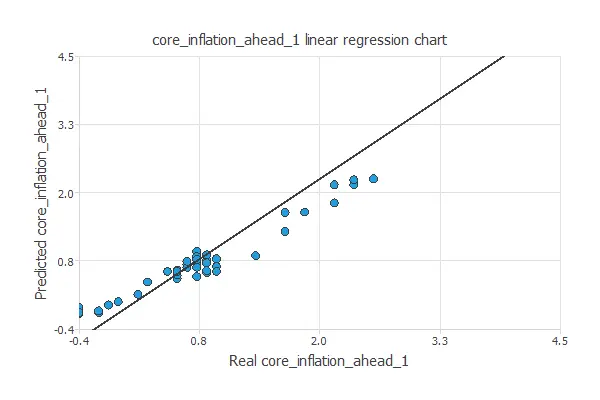The correlation value for this example is R2 = 0.977, which is close to 1. This means that we have a good predictive model.

We can also calculate the error statistics. The mean absolute error obtained by using the previous value as the prediction is 0.320. Using the model, it lowers to 0.186. Therefore, we are improving the prediction of the core inflation.

The final testing method we will use is the outputs plot. This will plot the real values (blue) and the predicted values (orange) over time.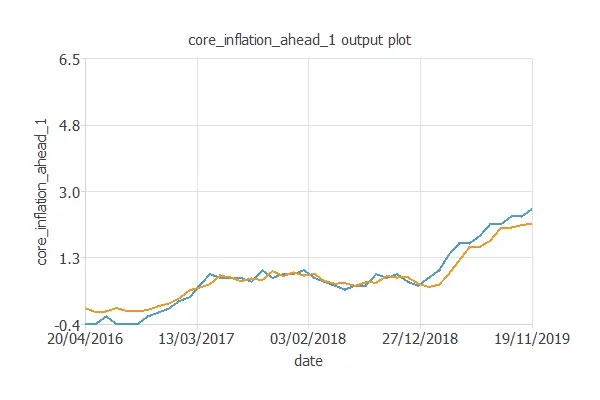## 7. Model deployment

The next listing shows the mathematical expression of the predictive model.

scaled_consumer_price_index_lag_2 = (consumer_price_index_lag_2-2.143300056)/1.813670039;
scaled_core_inflation_lag_2 = (core_inflation_lag_2-1.542860031)/1.364359975;
scaled_consumer_price_index_lag_1 = (consumer_price_index_lag_1-2.121880054)/1.779330015;
scaled_core_inflation_lag_1 = (core_inflation_lag_1-1.52410996)/1.32277;
scaled_consumer_price_index_lag_0 = (consumer_price_index_lag_0-2.10345006)/1.753630042;
scaled_core_inflation_lag_0 = (core_inflation_lag_0-1.507590055)/1.288020015;

perceptron_layer_1_output_00 = tanh( -0.42611 + (scaled_consumer_price_index_lag_2*-0.0133176) + (scaled_core_inflation_lag_2*-0.0242241) + (scaled_consumer_price_index_lag_1*-0.0445507) + (scaled_core_inflation_lag_1*0.0669062) + (scaled_consumer_price_index_lag_0*0.119317) + (scaled_core_inflation_lag_0*0.287741) );

perceptron_layer_2_output_00 = ( 1.14009 + (perceptron_layer_1_output_0*3.096) );

unscaling_layer_output_0 = -0.400000006+0.5*(perceptron_layer_2_output_0+1)*(6.5+0.400000006);



This formula can also be exported to the software tool the company requires.

## Conclusions

In this post, we have built a machine learning model to predict the inflation of a country.

## References

• The data for this problem has been taken from Kaggle.